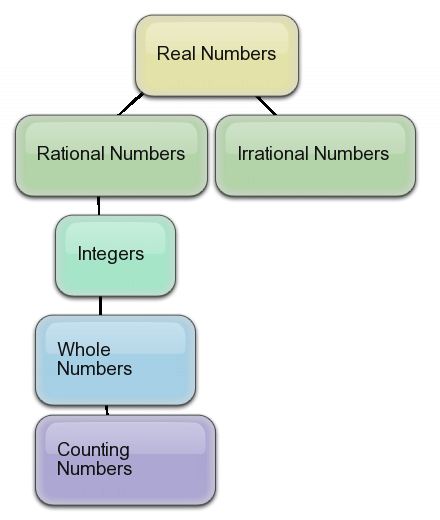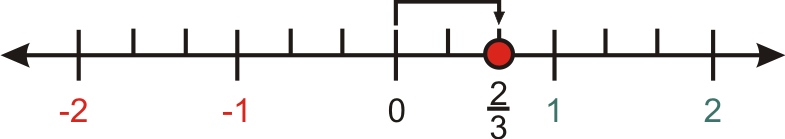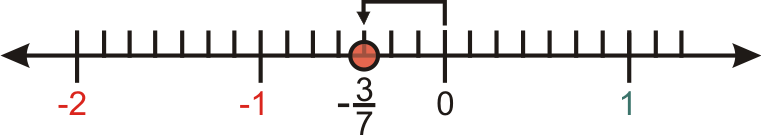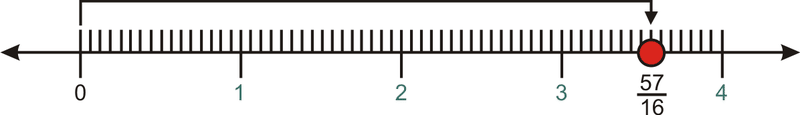# 1.2: Order of Real Numbers

•• Contributed by CK12
• CK12

## Order of Real Numbers

Suppose that you and three friends were playing a game where you each drew a number from a hat and the person with the highest number won. Let's say that you drew the number 32, while your friends drew the numbers 3–√, 1.7, and π3, respectively. Could you figure out who won the game?

### Ordering Real Numbers

#### Classifying Real Numbers[Figure 1]: The chart above shows the relationships between different real numbers. If a number is in one category, it is also in all the categories connected above it.

#### Let's use the chart to categorize the following numbers:

1. 0

Zero is a whole number, an integer, a rational number, and a real number.

1. –1

–1 is an integer, a rational number, and a real number.

1. π/3

π/3 is an irrational number and a real number.

1. √36/9

√36/9=6/9=2/3. This is a rational number and a real number.

#### Graphing and Ordering Real Numbers

Every real number can be positioned between two integers. Many times you will need to organize real numbers to determine the least value, greatest value, or both. This is usually done on a number line.

#### Let's plot the following rational numbers on a number line:

1. 2/3

2/3=0.667, which is between 0 and 1.[Figure 2]

1. −3/7

−3/7 is between –1 and 0.[Figure 3]

1. 57/16

57/16=3.5625#### Now, let's compare π/15 and √3/√75:

First we simplify in order to better compare:

√3/√75=√3/(5√3)=1/5.

Now we rewrite π/15 to compare it to 1/5:

π/15=π/(3×5)=π/3×1/5.

Since π>3,

π/3>1

so

π/3×1/5>1/5.

Therefore, π/15>√3/√75.

### Examples

Example 1 $$\PageIndex{1}$$

Earlier, you were asked to determine which number out of 3/2, √3, 1.7, and π/3 is the highest.

Solution

Notice that 3/2 = 1.5.

Also, since π≈3.14, π/3≈1.

Using a calculator, √3≈1.73.

Thus the order of the numbers is π/3 < 3/2 < 1.7 < √3 and √3 is the largest number.

Example 2 $$\PageIndex{1}$$

For the numbers: √12, (1.5)⋅(√3), 3/2, 2√5/√20, classify each number.

Solution

We need to simplify the numbers in order to classify them:

√12 = √((4x3)/2) = (2√3)/2 = √3. This is an irrational number. An irrational numbers is a type of real number.

(1.5)⋅(√3). This number cannot be simplified, but since it is a multiple of an irrational number, it is also irrational. In other words, we cannot get rid of the irrational part, and so we cannot write it as a rational number. It is also a real number.

3/2. Since this number is in the form of a proper fraction, it is also a rational number and real number.

(2√5)/√20 = (2√5)/(√4x5) = (2√5)/(2√5) = 1. This number can be simplified to an integer. All integers can be expressed as rational numbers and are a special kind of real number.

Example 3 $$\PageIndex{1}$$

For the numbers: √(12)/2, (1.5)⋅(√3), 3/2, (2√5)/√20, order the four numbers.

Solution

The four numbers are ordered as follows: 1<3/2<√3<(1.5)⋅√3.

1<3/2 since the numerator is larger then the denominator and 3/2=1.5.

3/2<√3 since we can see on our calculators that √3≈1.7

√3<(1.5)⋅√3 since multiplying by 1.5 makes any number larger.

### Review

Classify the following numbers. Include all the categories that apply to the number.

1. √0.25
2. √1.35
3. √20
4. √25
5. √100
6. Place the following numbers in numerical order from lowest to highest. 6–√261501.5−−−√1613
7. Find the value of each marked point;

Mixed Review

1. Simplify (9/4)÷6.
2. The area of a triangle is given by the formula A=b(h)/2, where b= base of the triangle and h= height of the triangle. Determine the area of a triangle with base =3 feet and height =7 feet.
3. Reduce the fraction 144/6.
4. Construct a table for the following situation: Tracey jumps 60 times per minute. Let the minutes be {0,1,2,3,4,5,6}. What is the range of this function?